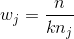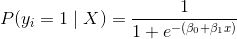# 十二、逻辑回归

## C 超参数快速调优

scikit-learn 的`LogisticRegressionCV`方法包含一个参数`C`。 如果提供了一个列表，`C`是可供选择的候选超参数值。 如果提供了一个整数，`C`的这么多个候选值，将从 0.0001 和 10000 之间的对数标度（`C`的合理值范围）中提取。

``````# 加载库
from sklearn import linear_model, datasets

# 加载数据
X = iris.data
y = iris.target

# 创建逻辑回归的交叉验证
clf = linear_model.LogisticRegressionCV(Cs=100)

# 训练模型
clf.fit(X, y)

'''
LogisticRegressionCV(Cs=100, class_weight=None, cv=None, dual=False,
fit_intercept=True, intercept_scaling=1.0, max_iter=100,
multi_class='ovr', n_jobs=1, penalty='l2', random_state=None,
refit=True, scoring=None, solver='lbfgs', tol=0.0001, verbose=0)
'''
``````

# 在逻辑回归中处理不平衡类别``````# 加载库
from sklearn.linear_model import LogisticRegression
from sklearn import datasets
from sklearn.preprocessing import StandardScaler
import numpy as np

# 加载数据
X = iris.data
y = iris.target

# 通过移除前 40 个观测，使类高度不均衡
X = X[40:,:]
y = y[40:]

# 创建目标向量，如果表示类别是否为 0
y = np.where((y == 0), 0, 1)

# 标准化特征
scaler = StandardScaler()
X_std = scaler.fit_transform(X)

# 创建决策树分类器对象
clf = LogisticRegression(random_state=0, class_weight='balanced')

# 训练模型
model = clf.fit(X_std, y)
``````

## 逻辑回归``````# 加载库
from sklearn.linear_model import LogisticRegression
from sklearn import datasets
from sklearn.preprocessing import StandardScaler

# 加载只有两个类别的数据
X = iris.data[:100,:]
y = iris.target[:100]

# 标准化特征
scaler = StandardScaler()
X_std = scaler.fit_transform(X)

# 创建逻辑回归对象
clf = LogisticRegression(random_state=0)

# 训练模型
model = clf.fit(X_std, y)

# 创建新的观测
new_observation = [[.5, .5, .5, .5]]

# 预测类别
model.predict(new_observation)

# array()

# 查看预测的概率
model.predict_proba(new_observation)

# array([[ 0.18823041,  0.81176959]])
``````

# 大量数据上的逻辑回归

scikit-learn 的`LogisticRegression`提供了许多用于训练逻辑回归的技术，称为求解器。 大多数情况下，scikit-learn 会自动为我们选择最佳求解器，或警告我们，你不能用求解器做一些事情。 但是，我们应该注意一个特殊情况。

``````# 加载库
from sklearn.linear_model import LogisticRegression
from sklearn import datasets
from sklearn.preprocessing import StandardScaler

# 加载数据
X = iris.data
y = iris.target

# 标准化特征
scaler = StandardScaler()
X_std = scaler.fit_transform(X)

# 创建使用 SAG 求解器的逻辑回归
clf = LogisticRegression(random_state=0, solver='sag')

# 训练模型
model = clf.fit(X_std, y)
``````

# 带有 L1 正则化的逻辑回归

L1 正则化（也称为最小绝对误差）是数据科学中的强大工具。 有许多教程解释 L1 正则化，我不会在这里尝试这样做。 相反，本教程将展示正则化参数`C`对系数和模型精度的影响。

``````import numpy as np
from sklearn.linear_model import LogisticRegression
from sklearn import datasets
from sklearn.model_selection import train_test_split
from sklearn.preprocessing import StandardScaler
``````

``````# 加载鸢尾花数据集

# 从特征中创建 X
X = iris.data

# 从目标中创建 y
y = iris.target

# 重新生成变量，保留所有标签不是 2 的数据
X = X[y != 2]
y = y[y != 2]

# 查看特征
X[0:5]

'''
array([[ 5.1,  3.5,  1.4,  0.2],
[ 4.9,  3\. ,  1.4,  0.2],
[ 4.7,  3.2,  1.3,  0.2],
[ 4.6,  3.1,  1.5,  0.2],
[ 5\. ,  3.6,  1.4,  0.2]])
'''

# 查看目标数据
y

'''
array([0, 0, 0, 0, 0, 0, 0, 0, 0, 0, 0, 0, 0, 0, 0, 0, 0, 0, 0, 0, 0, 0, 0,
0, 0, 0, 0, 0, 0, 0, 0, 0, 0, 0, 0, 0, 0, 0, 0, 0, 0, 0, 0, 0, 0, 0,
0, 0, 0, 0, 1, 1, 1, 1, 1, 1, 1, 1, 1, 1, 1, 1, 1, 1, 1, 1, 1, 1, 1,
1, 1, 1, 1, 1, 1, 1, 1, 1, 1, 1, 1, 1, 1, 1, 1, 1, 1, 1, 1, 1, 1, 1,
1, 1, 1, 1, 1, 1, 1, 1])
'''

# 将数据拆分为测试和训练集
# 将 30% 的样本放入测试集
X_train, X_test, y_train, y_test = train_test_split(X, y, test_size=0.3, random_state=0)
``````

``````# 创建缩放器对象
sc = StandardScaler()

# 将缩放器拟合训练数据，并转换
X_train_std = sc.fit_transform(X_train)

# 将缩放器应用于测试数据
X_test_std = sc.transform(X_test)
``````

L1 的用处在于它可以将特征系数逼近 0，从而创建一种特征选择方法。 在下面的代码中，我们运行带有 L1 惩罚的逻辑回归四次，每次都减少了`C`的值。 我们应该期望随着`C`的减少，更多的系数变为 0。

``````C = [10, 1, .1, .001]

for c in C:
clf = LogisticRegression(penalty='l1', C=c)
clf.fit(X_train, y_train)
print('C:', c)
print('Coefficient of each feature:', clf.coef_)
print('Training accuracy:', clf.score(X_train, y_train))
print('Test accuracy:', clf.score(X_test, y_test))
print('')

'''
C: 10
Coefficient of each feature: [[-0.0855264  -3.75409972  4.40427765  0\.        ]]
Training accuracy: 1.0
Test accuracy: 1.0

C: 1
Coefficient of each feature: [[ 0\.         -2.28800472  2.5766469   0\.        ]]
Training accuracy: 1.0
Test accuracy: 1.0

C: 0.1
Coefficient of each feature: [[ 0\.         -0.82310456  0.97171847  0\.        ]]
Training accuracy: 1.0
Test accuracy: 1.0

C: 0.001
Coefficient of each feature: [[ 0\.  0\.  0\.  0.]]
Training accuracy: 0.5
Test accuracy: 0.5
'''
``````

# OVR 逻辑回归

``````# 加载库
from sklearn.linear_model import LogisticRegression
from sklearn import datasets
from sklearn.preprocessing import StandardScaler

# 加载数据
X = iris.data
y = iris.target

# 标准化特征
scaler = StandardScaler()
X_std = scaler.fit_transform(X)

# 创建 OVR 逻辑回归对象
clf = LogisticRegression(random_state=0, multi_class='ovr')

# 训练模型
model = clf.fit(X_std, y)

# 创建新的观测
new_observation = [[.5, .5, .5, .5]]

# 预测类别
model.predict(new_observation)

# array()

# 查看预测概率
model.predict_proba(new_observation)

# array([[ 0.0829087 ,  0.29697265,  0.62011865]])
``````